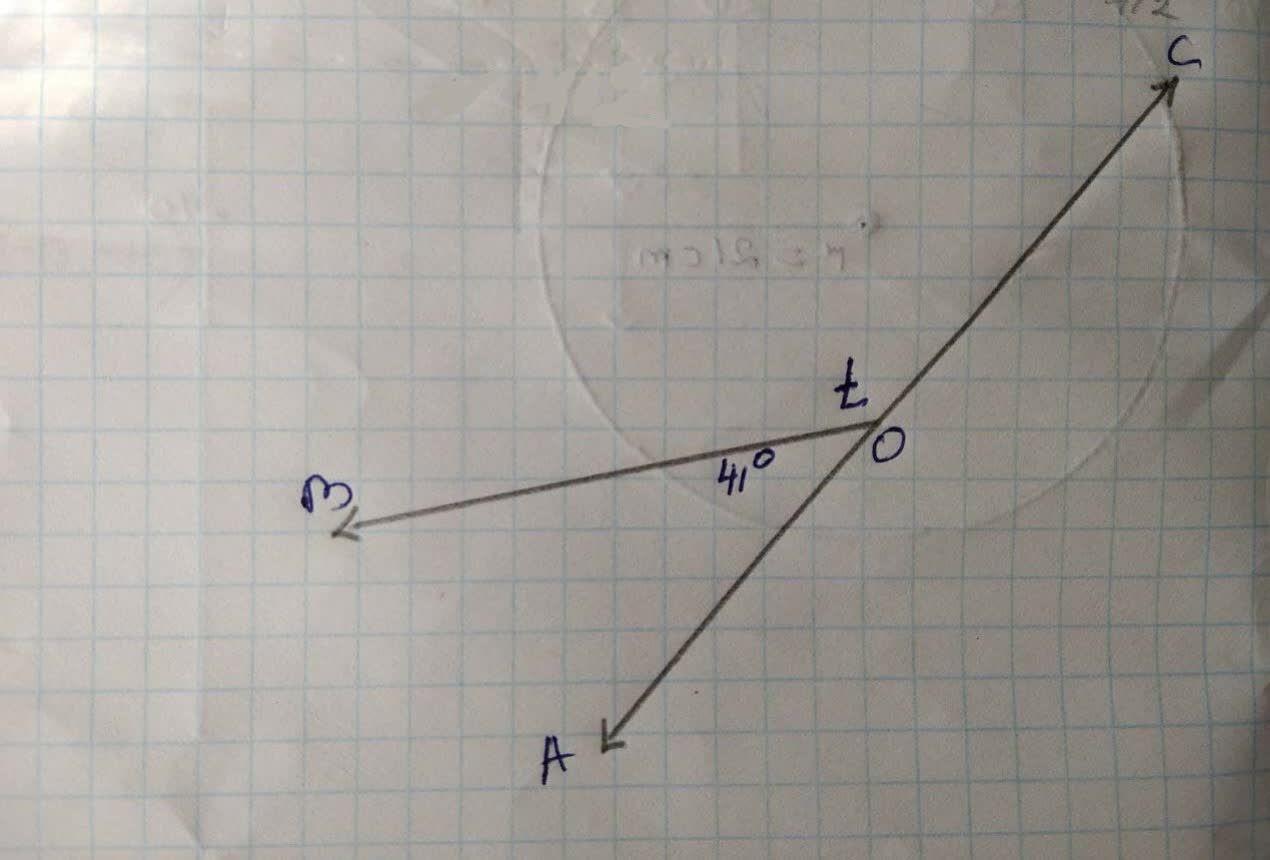# What is the value of t? FSP19610807861.jpgFSZJason Watson 2021-11-28 Answered
What is the value of t?You can still ask an expert for help

• Questions are typically answered in as fast as 30 minutes

Solve your problem for the price of one coffee

• Math expert for every subject
• Pay only if we can solve itMelinda Olson

Given figurc
We find thc volue of t
From thc figure we sec thc t
$\mathrm{\angle }AOB+\mathrm{\angle }BOC=180°$

$⇒41°+t=180°$
$\left[\therefore \mathrm{\angle }AOB=41°\mathrm{\angle }BOC=t\right]$
$⇒t=180°-41°$
$⇒t=139°$
Hence $t=139°$

###### Not exactly what you’re looking for?Jeffrey Jordon